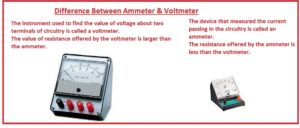Hello, friends, I hope you all are doing great. In today’s tutorial, we will discuss the Difference Between Ammeter & Voltmeter. The basic difference between ammeter and voltmeter is that ammeter is used to find the value of current flowing in the circuitry and voltmeter is used to measure the value of the voltage at 2 terminals of circuitry. There are 2 steps to find the value of electricity first through current second one through voltage. The current is calculated with the ammeter and voltage measured with the voltmeter.

The working operation of both ammeter and voltmeter is similar to the operation of a galvanometer. In the galvanometer there is the coil is placed between a permanent magnet. After the flow of current in the coil gets deflected. The coil deflection depends on the current flowing through the coil. The deflection of coil helps to find the value of current and voltage. If resistance is connected in series combination with the galvanometer it operates as ammeter. In today’s post, we will have a detailed look at both ammeter and voltmeter and compare them to find their differences. So let’s get started with Difference Between Ammeter & Voltmeter.

#### Difference Between Ammeter & Voltmeter

Ammeter

• The device that measured the current passing in the circuitry is called an ammeter.
• The resistance offered by the ammeter is less than the voltmeter.
• It should link to supply since it has less value of resistance and can be damaged due to large current flow.
• Some meter has a range of a few mA of currents.
• For increase, the measuring range of the meter there should be resistance called shunt show connected to ammeter ins parallel combination.
• It normally has a feature to operates for less voltage range normally single volts or less
• The circuit for which the current is measured ammeter is attached in a series combination.
• Its accuracy is larger than the voltmeter.
• Its range can not be varied.Voltmeter

• The instrument used to find the value of voltage about two terminals of circuitry is called a voltmeter.
• The value of resistance offered by the voltmeter is larger than the ammeter.
• Its resistance value is large than the ammeter.
• Analog meter has a needle that indicates the voltage value that to be calculated.
• To measure the small value of voltage it linked to amplifier circuitry.
• Digital meter indicates voltage in numbers
• The circuit about which voltage is measured voltmeter is connected in parallel combination.
• It is less accurate than the ammeter.
• Its measuring range can be varied.
• Some meter gets power from an outer source and some have its own power source to operate.
• With transformer different voltmeter used to show the value of volts at input and output.
• For measuring the volts value from some volts to thousand volts general-purpose voltmeter used
• Digital meter has a high level of correctness normally one percent than other

That is a detailed post about the difference between ammeter and a voltmeter. If you have any query ask in comments. Thanks for reading. Have a good day.